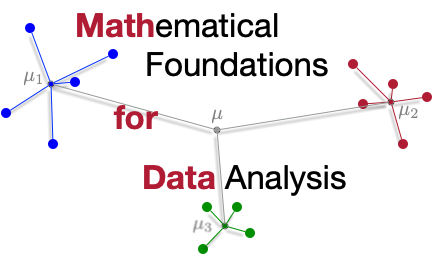# Math for DataMathematical Foundations for Data Analysis

# Errata

Send information on any mistakes found to mathfordata /at/ gmail.com

• pages 73, 151, 244 : A code block invokes “SciPy” when it should invoke “scipy” with all lower case letters.

• page 154 : For matrices U, V from the SVD the book states the Frobenious norm of these matrices is 1 (that is, ||U||_F=1 and ||V||_F=1). It should state the spectral norm is 1 (that is, ||U||_2=1 and ||V||_2=1), and point out they are norm-preserving under multiplication. h/t David R. Gurney

• page 163 : Let E_i be an nxn matrix that is all 0s except the ith column which is all 1s, and Z an nxn matrix. The books states E_i Z has each column as the ith column of Z, and Z E_i^T has each row as the ith row of Z. It should almost be the reverse where E_i Z has each row as the ith row of Z, and Z E_i^T has each row as the ith column of Z. h/t David R. Gurney